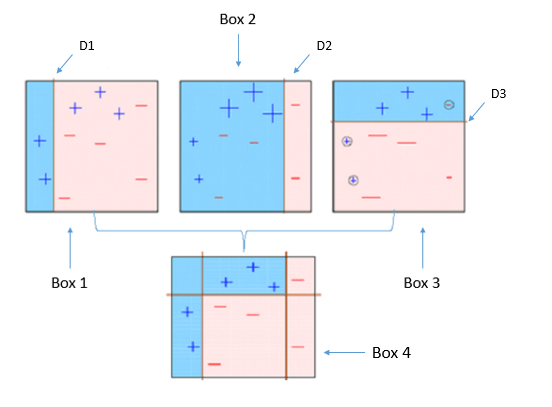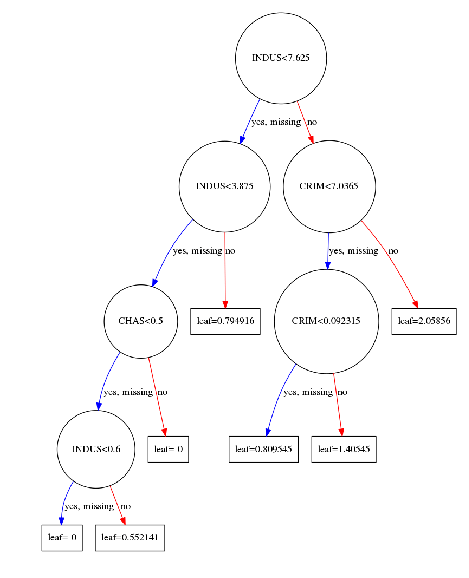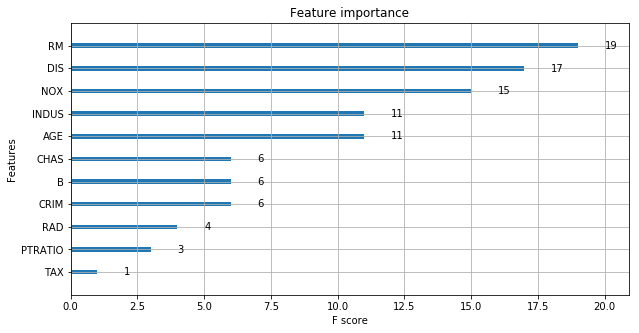source:https://www.datacamp.com/community/tutorials/xgboost-in-python

XGBoost is one of the most popular machine learning algorithm these days. Regardless of the type of prediction task at hand; regression or classification.

XGBoost is well known to provide better solutions than other machine learning algorithms. In fact, since its inception, it has become the “state-of-the-art” machine learning algorithm to deal with structured data.

In this tutorial, you’ll learn to build machine learning models using XGBoost in python. More specifically you will learn:

But what makes XGBoost so popular?

• Speed and performance : Originally written in C++, it is comparatively faster than other ensemble classifiers.
• Core algorithm is parallelizable : Because the core XGBoost algorithm is parallelizable it can harness the power of multi-core computers. It is also parallelizable onto GPU’s and across networks of computers making it feasible to train on very large datasets as well.
• Consistently outperforms other algorithm methods : It has shown better performance on a variety of machine learning benchmark datasets.
• Wide variety of tuning parameters : XGBoost internally has parameters for cross-validation, regularization, user-defined objective functions, missing values, tree parameters, scikit-learn compatible API etc.

XGBoost (Extreme Gradient Boosting) belongs to a family of boosting algorithms and uses the gradient boosting (GBM) framework at its core. It is an optimized distributed gradient boosting library. But wait, what is boosting? Well, keep on reading.

## Boosting

Boosting is a sequential technique which works on the principle of an ensemble. It combines a set of weak learners and delivers improved prediction accuracy. At any instant t, the model outcomes are weighed based on the outcomes of previous instant t-1. The outcomes predicted correctly are given a lower weight and the ones miss-classified are weighted higher. Note that a weak learner is one which is slightly better than random guessing. For example, a decision tree whose predictions are slightly better than 50%. Let’s understand boosting in general with a simple illustration.Four classifiers (in 4 boxes), shown above, are trying to classify + and - classes as homogeneously as possible.

1. Box 1: The first classifier (usually a decision stump) creates a vertical line (split) at D1. It says anything to the left of D1 is + and anything to the right of D1 is -. However, this classifier misclassifies three + points.

Note a Decision Stump is a Decision Tree model that only splits off at one level, therefore the final prediction is based on only one feature.

2. Box 2: The second classifier gives more weight to the three + misclassified points (see the bigger size of +) and creates a vertical line at D2. Again it says, anything to the right of D2 is - and left is +. Still, it makes mistakes by incorrectly classifying three - points.

3. Box 3: Again, the third classifier gives more weight to the three - misclassified points and creates a horizontal line at D3. Still, this classifier fails to classify the points (in the circles) correctly.

4. Box 4: This is a weighted combination of the weak classifiers (Box 1,2 and 3). As you can see, it does a good job at classifying all the points correctly.

That’s the basic idea behind boosting algorithms is building a weak model, making conclusions about the various feature importance and parameters, and then using those conclusions to build a new, stronger model and capitalize on the misclassification error of the previous model and try to reduce it. Now, let’s come to XGBoost. To begin with, you should know about the default base learners of XGBoost: tree ensembles. The tree ensemble model is a set of classification and regression trees (CART). Trees are grown one after another ,and attempts to reduce the misclassification rate are made in subsequent iterations. Here’s a simple example of a CART that classifies whether someone will like computer games straight from the XGBoost’s documentation.

If you check the image in Tree Ensemble section, you will notice each tree gives a different prediction score depending on the data it sees and the scores of each individual tree are summed up to get the final score.

In this tutorial, you will be using XGBoost to solve a regression problem. The dataset is taken from the UCI Machine Learning Repository and is also present in sklearn’s datasetsmodule. It has 14 explanatory variables describing various aspects of residential homes in Boston, the challenge is to predict the median value of owner-occupied homes per $1000s. ## Using XGBoost in Python First of all, just like what you do with any other dataset, you are going to import the Boston Housing dataset and store it in a variable called boston. To import it from scikit-learn you will need to run this snippet. from sklearn.datasets import load_boston boston = load_boston()  The boston variable itself is a dictionary, so you can check for its keys using the .keys()method. print(boston.keys())  dict_keys(['data', 'target', 'feature_names', 'DESCR'])  You can easily check for its shape by using the boston.data.shape attribute, which will return the size of the dataset. print(boston.data.shape)  (506, 13)  As you can see it returned (506, 13), that means there are 506 rows of data with 13 columns. Now, if you want to know what the 13 columns are, you can simply use the .feature_namesattribute and it will return the feature names. print(boston.feature_names)  ['CRIM' 'ZN' 'INDUS' 'CHAS' 'NOX' 'RM' 'AGE' 'DIS' 'RAD' 'TAX' 'PTRATIO' 'B' 'LSTAT']  The description of the dataset is available in the dataset itself. You can take a look at it using .DESCR. print(boston.DESCR)  Boston House Prices dataset =========================== Notes ------ Data Set Characteristics: :Number of Instances: 506 :Number of Attributes: 13 numeric/categorical predictive :Median Value (attribute 14) is usually the target :Attribute Information (in order): - CRIM per capita crime rate by town - ZN proportion of residential land zoned for lots over 25,000 sq.ft. - INDUS proportion of non-retail business acres per town - CHAS Charles River dummy variable (= 1 if tract bounds river; 0 otherwise) - NOX nitric oxides concentration (parts per 10 million) - RM average number of rooms per dwelling - AGE proportion of owner-occupied units built prior to 1940 - DIS weighted distances to five Boston employment centres - RAD index of accessibility to radial highways - TAX full-value property-tax rate per$10,000
- PTRATIO  pupil-teacher ratio by town
- B        1000(Bk - 0.63)^2 where Bk is the proportion of blacks by town
- LSTAT    % lower status of the population

## k-fold Cross Validation using XGBoost

In order to build more robust models, it is common to do a k-fold cross validation where all the entries in the original training dataset are used for both training as well as validation. Also, each entry is used for validation just once. XGBoost supports k-fold cross validation via the cv() method. All you have to do is specify the nfolds parameter, which is the number of cross validation sets you want to build. Also, it supports many other parameters (check out this link) like:

• num_boost_round: denotes the number of trees you build (analogous to n_estimators)
• metrics: tells the evaluation metrics to be watched during CV
• as_pandas: to return the results in a pandas DataFrame.
• early_stopping_rounds: finishes training of the model early if the hold-out metric (“rmse” in our case) does not improve for a given number of rounds.
• seed: for reproducibility of results.

This time you will create a hyper-parameter dictionary params which holds all the hyper-parameters and their values as key-value pairs but will exclude the n_estimators from the hyper-parameter dictionary because you will use num_boost_rounds instead.

You will use these parameters to build a 3-fold cross validation model by invoking XGBoost’s cv() method and store the results in a cv_results DataFrame. Note that here you are using the Dmatrix object you created before.

params = {"objective":"reg:linear",'colsample_bytree': 0.3,'learning_rate': 0.1,
'max_depth': 5, 'alpha': 10}

cv_results = xgb.cv(dtrain=data_dmatrix, params=params, nfold=3,
num_boost_round=50,early_stopping_rounds=10,metrics="rmse", as_pandas=True, seed=123)


cv_results contains train and test RMSE metrics for each boosting round.

cv_results.head()

test-rmse-mean test-rmse-std train-rmse-mean train-rmse-std
0 21.746693 0.019311 21.749371 0.033853
1 19.891096 0.053295 19.859423 0.029633
2 18.168509 0.014465 18.072169 0.018803
3 16.687861 0.037342 16.570206 0.018556
4 15.365013 0.059400 15.206344 0.015451

Extract and print the final boosting round metric.

print((cv_results["test-rmse-mean"]).tail(1))

49    4.031162
Name: test-rmse-mean, dtype: float64


You can see that your RMSE for the price prediction has reduced as compared to last time and came out to be around 4.03 per 1000\$. You can reach an even lower RMSE for a different set of hyper-parameters. You may consider applying techniques like Grid Search, Random Search and Bayesian Optimization to reach the optimal set of hyper-parameters.

## Visualize Boosting Trees and Feature Importance

You can also visualize individual trees from the fully boosted model that XGBoost creates using the entire housing dataset. XGBoost has a plot_tree() function that makes this type of visualization easy. Once you train a model using the XGBoost learning API, you can pass it to the plot_tree() function along with the number of trees you want to plot using the num_trees argument.

xg_reg = xgb.train(params=params, dtrain=data_dmatrix, num_boost_round=10)


Plotting the first tree with the matplotlib library:

import matplotlib.pyplot as plt

xgb.plot_tree(xg_reg,num_trees=0)
plt.rcParams['figure.figsize'] = [50, 10]
plt.show()These plots provide insight into how the model arrived at its final decisions and what splits it made to arrive at those decisions.

Note that if the above plot throws the ‘graphviz’ error on your system, consider installing the graphviz package via pip install graphviz on cmd. You may also need to run sudo apt-get install graphviz on cmd. (link)

Another way to visualize your XGBoost models is to examine the importance of each feature column in the original dataset within the model.

One simple way of doing this involves counting the number of times each feature is split on across all boosting rounds (trees) in the model, and then visualizing the result as a bar graph, with the features ordered according to how many times they appear. XGBoost has a plot_importance() function that allows you to do exactly this.

xgb.plot_importance(xg_reg)
plt.rcParams['figure.figsize'] = [5, 5]
plt.show()As you can see the feature RM has been given the highest importance score among all the features. Thus XGBoost also gives you a way to do Feature Selection. Isn’t this brilliant?

## Conclusion

You have reached the end of this tutorial. I hope this might have or will help you in some way or the other. You started off with understanding how Boosting works in general and then narrowed down to XGBoost specifically. You also practiced applying XGBoost on an open source dataset and along the way you learned about its hyper-parameters, doing cross-validation, visualizing the trees and in the end how it can also be used as a Feature Selection technique. Whoa!! that’s something for starters, but there is so much to explore in XGBoost that it can’t be covered in a single tutorial. If you would like to learn more, be sure to take a look at our Extreme Gradient Boosting with XGBoost course on DataCamp.

Updated: